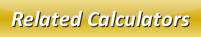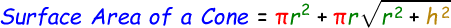# Surface Area of a Cone Calculator

We can find the total surface area of a cone when we add the base surface area of a cone and lateral surface area of a cone.

Formula to calculate surface area of a cone is given below:r = Radius of the cone
h = height of the cone

Find total surface area of a cone with the help of our below online surface area of a cone calculator. Enter the radius and height of the cone and click calculate to find the answer.

 Radius of the Cone: Height of the Cone: Surface Area of the Cone:

Latest Calculator Release

Average Acceleration Calculator

Average acceleration is the object's change in speed for a specific given time period. ...

Free Fall Calculator

When an object falls into the ground due to planet's own gravitational force is known a...

Torque Calculator

Torque is nothing but a rotational force. In other words, the amount of force applied t...

Average Force Calculator

Average force can be explained as the amount of force exerted by the body moving at giv...

Angular Displacement Calculator

Angular displacement is the angle at which an object moves on a circular path. It is de...